# Calling VBA and other Excel add-in functions¶

xlSlim makes it easy to use functions from VBA or other Excel add-ins in your Python code.

## Calling VBA functions¶

```Public Function AreaCircle(radius As Double)
AreaCircle = 2 * WorksheetFunction.Pi() * radius
End Function
```

The function must be defined in a VBA Module in the VBA editor (The VBA editor can be opened with Alt-F11 in Excel).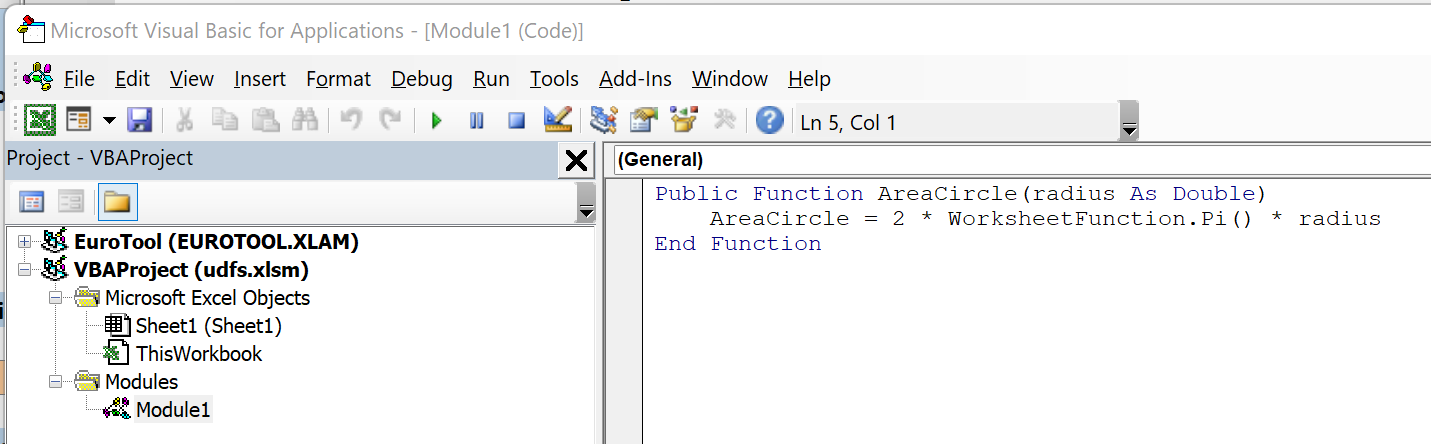This Python module accesses the VBA function. Note the global variable XLSLIM_UDF that is used to access VBA functions.

```# This global is used to store a function pointer set by xlSlim
XLSLIM_UDFFUNC = None

"""Calculate the area of a circle using the VBA function AreaCircle"""
```

Note

All the Python code and Excel files shown are available from github in the xlslim-code-samples repo. I highly recommend downloading the samples from github. The Excel workbooks contain many tips and tricks.

Save the Python code as a new file on your PC. I saved the file in my Documents folder.

Enter this `RegisterPyModule()` formula in Excel (amending the location to match where you saved the file):

```=RegisterPyModule("C:\Users\russe\Documents\udfs.py")
```

The function is now available to use in Excel.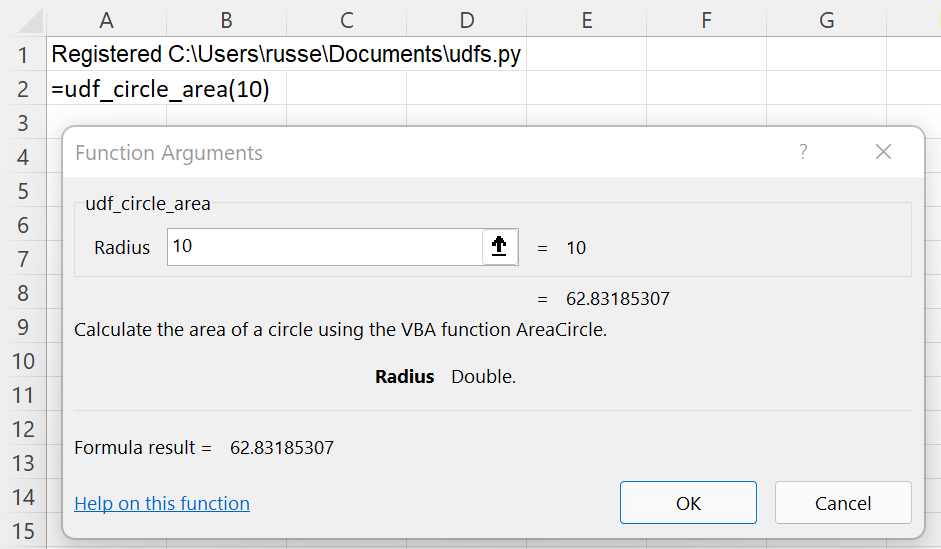As you would expect, the result is exactly the same as calling the VBA function directly in Excel.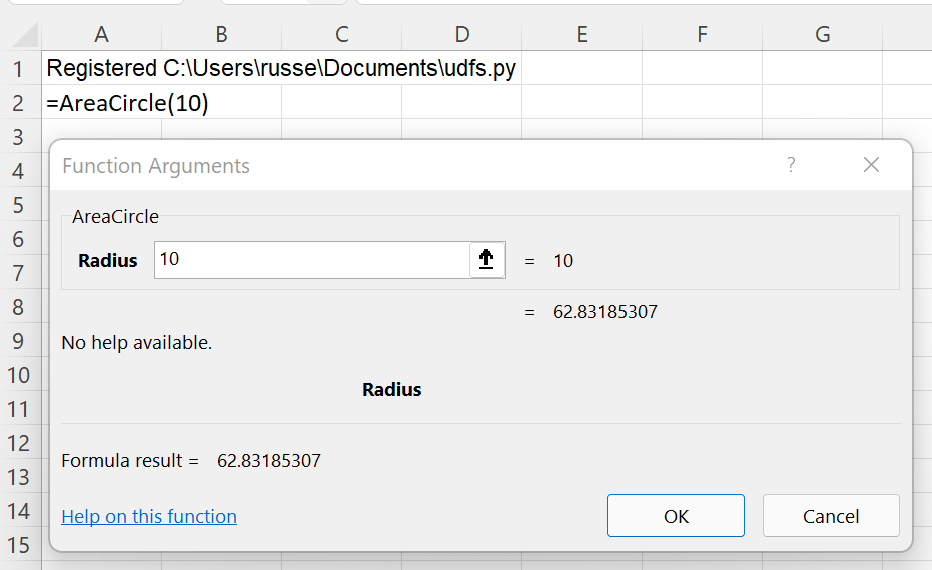The key is the global variable XLSLIM_UDF. Any function accessible to Excel can be called in Python using XLSLIM_UDF(<function name>, <arg 1>, <arg 2>…<arg n>)

## Calling other Excel add-in functions¶

Excel is distributed with an add-in to convert between the pre-unification currencies of Euro member countries. Install this add-in by following the instructions at EUROCONVERT_function

Once the add-in is installed it can be used to convert 100 Austrian schillings to French francs.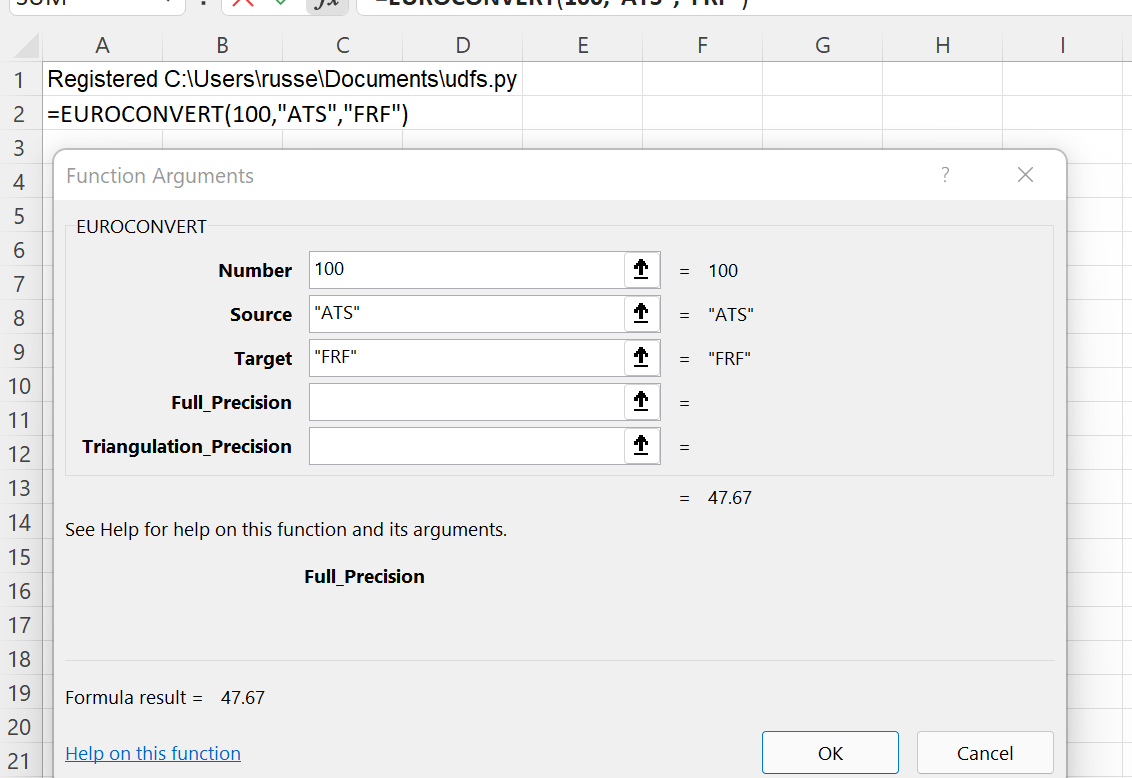Add the eur_conversion() function below to the Python module. The module should now contain:

```# This global is used to store a function pointer set by xlSlim
XLSLIM_UDFFUNC = None

"""Calculate the area of a circle using the VBA function AreaCircle"""

def eur_conversion(amount: float, from_currency: str, to_currency: str) -> float:
"""Convert to EUR using the Excel addin function EUROCONVERT."""
return XLSLIM_UDFFUNC("EUROCONVERT", amount, from_currency, to_currency)
```

Note

All the Python code and Excel files shown are available from github in the xlslim-code-samples repo. I highly recommend downloading the samples from github. The Excel workbooks contain many tips and tricks.

Reload the module in Excel (amending the location to match where you saved the file):

```=RegisterPyModule("C:\Users\russe\Documents\udfs.py")
```

The function is now available to use in Excel.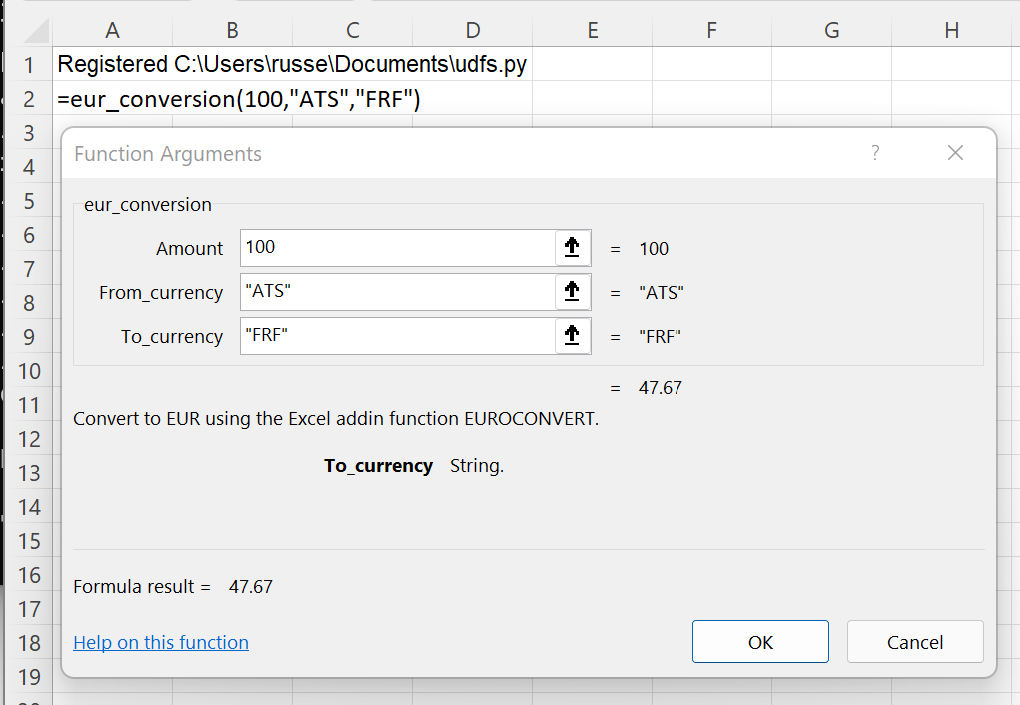As you would expect, the result is exactly the same as calling the add-in function directly in Excel.